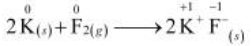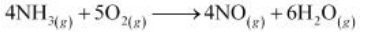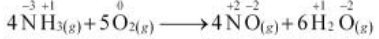The oxidation number of each element in the given reaction can be represented as:In this reaction, the oxidation number of K increases from 0 in K to +1 in KF i.e., K is oxidized to KF. On the other hand, the oxidation number of F decreases from 0 in F${}_{2}$ to –1 in KF i.e., F is reduced to KF.

Hence, the above reaction is a redox reaction.

(e)The oxidation number of each element in the given reaction can be represented as:Here, the oxidation number of N increases from –3 in NH to +2 in NO. On the other hand, the oxidation number of O decreases from 0 in O to –2 in NO and HO i.e., O is reduced. Hence, the given reaction is a redox reaction.

8.4 Fluorine reacts with ice and results in the change:

H2O(s) + F2(g) HF(g) + HOF(g)

Justify that this reaction is a redox reaction.

Let us write the oxidation number of each atom involved in the given reaction above its symbol as:

${\stackrel{+1}{\mathrm{H}}}_{2}\stackrel{-2}{O}+\stackrel{0}{{F}_{2}}\to \stackrel{+1}{H}\stackrel{-1}{F}+\stackrel{+1}{H}\stackrel{-2}{O}\stackrel{+1}{F}$

Here, we have observed that the oxidation number of F increases from 0 in ${F}_{2}$ to +1 in HOF. Also, the oxidation number decreases from 0 in ${F}_{2}$ to –1 in HF. Thus, in the above reaction, F is both oxidized and reduced. Hence, the given reaction is a redox reaction.